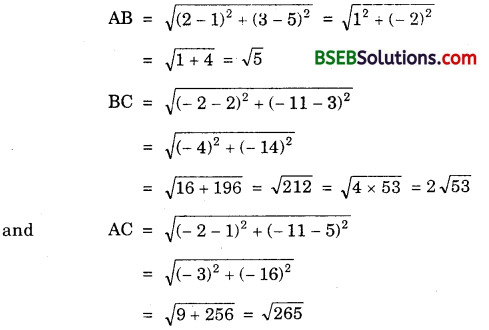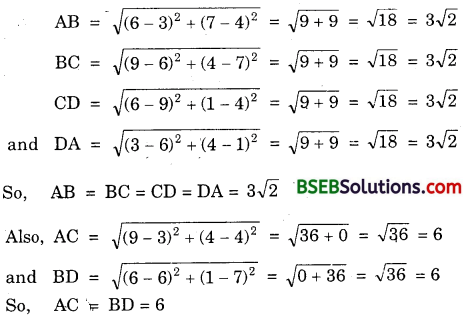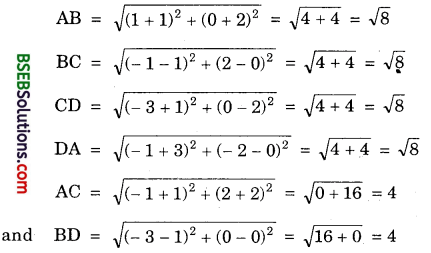# Bihar Board Class 10th Maths Solutions Chapter 7 Coordinate Geometry Ex 7.1

Bihar Board Class 10th Maths Solutions Chapter 7 Coordinate Geometry Ex 7.1 Textbook Questions and Answers.

## BSEB Bihar Board Class 10th Maths Solutions Chapter 7 Coordinate Geometry Ex 7.1Question 1.
Find the distance between the following pairs of points :
(i) (2, 3), (4, 1)
(ii) (- 5, 7), (- 1, 3)
(iii) (a, b), (- a, – b)
Solution:
(i) Let P(2, 3) and Q(4, 1) be the given points.
Here, x1 = 2, y1 = 3 and x2 = 4, y2 = 1.
∴ PQ = $$\sqrt{\left(x_{2}-x_{1}\right)^{2}+\left(y_{2}-y_{1}\right)^{2}}$$
So, PQ = $$\sqrt{(4-2)^{2}+(1-3)^{2}}$$ = $$\sqrt{(2)^{2}+(-2)^{2}}$$
or PQ = $$\sqrt{4+4}$$ = $$\sqrt{8}$$ = 2$$\sqrt{2}$$

(ii) Let P(- 5, 7) and Q(- 1, 3) be the given points.
Here, x1 = -5, y1 = 7 and x2 = -1, y2 = 3.
∴ PQ = $$\sqrt{\left(x_{2}-x_{1}\right)^{2}+\left(y_{2}-y_{1}\right)^{2}}$$
So, PQ = $$\sqrt{(-1+5)^{2}+(3-7)^{2}}$$ = $$\sqrt{(4)^{2}+(-4)^{2}}$$
or PQ = $$\sqrt{16+16}$$ = $$\sqrt{32}$$ = $$\sqrt{16 \times 2}$$ = 4$$\sqrt{2}$$

(iii) Let P(a, b) and Q(- a, – b) be the given points.
Here, x1 = a, y1 = b and x2 = a, y2 = – b.
∴ PQ = $$\sqrt{\left(x_{2}-x_{1}\right)^{2}+\left(y_{2}-y_{1}\right)^{2}}$$
So, PQ = $$\sqrt{(-a-a)^{2}+(-b-b)^{2}}$$
= $$\sqrt{(-2 a)^{2}+(-2 b)^{2}}$$
or PQ = $$\sqrt{4 a^{2}+4 b^{2}}$$
= 2$$\sqrt{a^{2}+b^{2}}$$Question 2.
Find the distance between the points (0, 0) and (36, 15). Can you now find the distance between the two towns A and B discussed in Section 7.2?
Solution:
Let P(0, 0) and Q(36, 15) be the given points.
Here, x1 = 0, y1 = 0 and x2 = 36, y2 = 15.
∴ PQ = $$\sqrt{\left(x_{2}-x_{1}\right)^{2}+\left(y_{2}-y_{1}\right)^{2}}$$
So, PQ = $$\sqrt{(36-0)^{2}+(15-0)^{2}}$$ = $$\sqrt{1296+225}$$
= $$\sqrt{1521}$$ = 39
In fact, the positions of towns A and B are given by (0, 0) and (36, 15) respectively and so the distance between them = 39 km as calculated above.

Question 3.
Determine if the points (1, 5), (2, 3) and (- 2, – 11) are collinear.
Solution:
Let A(1, 5), B(2, 3) and C(- 2, – 11) be the given points. Then, we have :Clearly, BC ≠ AB + AC, AB ≠ BC + AC and AC ≠ BC + AB.
Hence, A, B and C are not collinear.

Question 4.
Check whether (5, – 2), (6, 4) and (7, – 2) are the vertices of an isosceles triangle.
Solution:
Let A(5, – 2), B(6, 4) and C(7, – 2) are the given points. Then,
AB = $$\sqrt{(6-5)^{2}+(4+2)^{2}}$$
= $$\sqrt{1+36}$$
= $$\sqrt{37}$$
BC = $$\sqrt{(7-6)^{2}+(-2-4)^{2}}$$
= $$\sqrt{1+36}$$
= $$\sqrt{37}$$
Clearly, AB = BC
∴ ∆ ABC is an isosceles triangle.Question 5.
In a classroom, four friends are Seated at the points A, B, C and D as shown in figure. Champa and Chameli walk into the class and after observing for a few minutes Champa asks Chameli, “Don’t you think ABCD is a square ?” Chameli disagrees. Using distance formula, find which of them is correct, and why?
Solution:
Clearly from the figure, the coordinates of points A, B, C and D are (3, 4), (6, 7), (9, 4) and (6, 1).
By using distance formula, we getThus, the four sides are equal and the diagonals are also equal. Therefore, ABCD is a square.
Hence, Champa is correct.

Question 6.
Name the type of quadrilateral formed, if any, by the following points, and give reasons for your answer :
(i) (- 1, – 2), (1, 0), (- 1, 2), (- 3, 0)
(ii) (-3, 5), (3, 1), (0, 3), (- 1,-4)
(iii) (4, 5), (7, 6), (4, 3), (1, 2)
Solution:
(i) Let A(- 1, – 2), B(1, 0), C(- 1, 2) and D(- 3, 0) be the given points. Then,Clearly, four sides AB, BC, CD and DA are equal.
Also, diagonals AC and BD are equal.
∴ The quadrilateral ABCD is a square.

(ii) Let A(- 3, 5), B(3, 1), C(0. 3) and D(- 1, – 4) be the given points. Plot these points as shown.Clearly, the points A, C and B are collinear.
So, no quadrilateral is formed by these points.Aliter :
AB = $$\sqrt{(3+3)^{2}+(1-5)^{2}}$$
= $$\sqrt{36+16}$$
= $$\sqrt{52}$$
= 2$$\sqrt{13}$$

BC = $$\sqrt{(3-0)^{2}+(1-3)^{2}}$$
and AC = $$\sqrt{(0+3)^{2}+(3-5)^{2}}$$ = $$\sqrt{13}$$
So, AC = AB + BC.
Hence, there, will not be any quadrilateral.

(iii) Let A(4, 5), B(7, 6), C(4, 3) and D(1, 2) be the .given points. Then,Clearly, AB = CD, BC = DA and AC = BD.
∴ The quadrilateral ABCD is a parallelogram.Question 7.
Find the point on the x-axis which is equidistant from (2, – 5) and (- 2, 9).
Solution:
Since the point on x-axis have its ordinate – 0, so P(x, 0) is any point on the x-axis.
Since P(x, 0) is equidistant from A(2, – 5) and P (- 2, 9), therefore PA = PB gives. PA² = PB²
or (x – 2)² + (0 + 5)² = (x + 2)² + (0 – 9)²
or x² – 4x + 4 + 25 = x² + 4x + 4 + 81
or – 4x – 4x = 81 – 25
– 8x = 56
or x = $$\frac { 56 }{ -8 }$$ = – 7
∴ The point equidistant from given points on the x-axis is (- 7, 0).

Question 8.
Find the values of y for which the distance between the points P(2, – 3) and Q(10, y) is 10 units.
Solution:
P(2, – 3) and Q(10, y) are given points such that PQ = 10 units.Thus, the possible value of y is – 9 or 3.

Question 9.
If Q(0, 1) is equidistant from P(5, – 3) and R(x, 6), find the values of x. Also, find the distances QR and PR.
Solution:
Since the point Q(0, 1) is equidistant from P(5, – 3) and R(x, 6), therefore
QP = QR gives QP² – QR²
or (5 – 0)² + (- 3 – 1)² = (x – 0)² + (6 – 1)²
or 25 + 16 = x² + 25
or x² = 16 So, x = ± 4
Thus, R is (4, 6) or (- 4, 6).
Now, QR = Distance between Q(0, 1) and R(4, 6)
$$\sqrt{(4-0)^{2}+(6-1)^{2}}$$ = $$\sqrt{16+25}$$ = $$\sqrt{41}$$
Also, QR = Distance between Q(0, 1) and R(- 4, 6)
$$\sqrt{(-4-0)^{2}+(6-1)^{2}}$$ = $$\sqrt{16+25}$$ = $$\sqrt{41}$$
and PR = Distance between P(5, – 3) and R(4, 6)
$$\sqrt{(4-5)^{2}+(6+3)^{2}}$$ = $$\sqrt{1+81}$$ = $$\sqrt{82}$$
and PR = Distance between P(5, – 3) and R(-4, 6)
$$\sqrt{(-4-5)^{2}+(6+3)^{2}}$$ = $$\sqrt{81+81}$$ = 9$$\sqrt{2}$$Question 10.
Find a relation between x and y such that the point Or, y) is equidistant from the points (3, 6) and (-3,4).
Solution:
Let the point P(x, y) be equidistant from the points A(3, 6) and B(- 3, 4).
i.e., PA = PB gives PA² = PB²
or (x – 3)² + (y – 6)² = (x + 3)² + (y – 4)²
or x²- 6x + 9 + y² – 12y + 36 = x²+ 6x + 9 + y² – 8y + 16
or 6x – 6x – 12y + 8y + 36 – 16 0
or – 12x – 4y + 20 = 0
or 3x + y – 5 = 0,
which is the required relation.